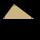# Triangle + arithmetic progression - math problems

#### Number of problems found: 11

• The perimeterThe perimeter of the triangle is 24, the sides are integers and form an arithmetic sequence. Specify the side sizes of this triangle.
• The sidesThe sides of a right triangle form an arithmetic sequence. The hypotenuse is 24 cm long. Determine the remaining sides of the triangle.
• Rectangular triangleThe lengths of the rectangular triangle sides with a longer leg of 12 cm form an arithmetic sequence. What is the area of the triangle?
• Infinite sum of areasAbove the height of the equilateral triangle ABC is constructed an equilateral triangle A1, B1, C1, of the height of the equilateral triangle built A2, B2, C2, and so on. The procedure is repeated continuously. What is the total sum of the areas of all tr
• AP RT triangleThe length of the sides of a right triangle forms an arithmetic progression, and the longer leg is 24 cm long. What are the perimeter and area?
• Right-angled triangleDetermine the content of a right triangle whose side lengths form successive members of an arithmetic progression and the radius of the circle described by the triangle is 5 cm.
• Angle in RTDetermine the size of the smallest internal angle of a right triangle whose sides constitutes sizes consecutive members of arithmetic progressions.
• AngleDetermine the size of the smallest internal angle of a right triangle which angles forming the successive members of the arithmetic sequence.
• Angles in a triangleThe angles of the triangle ABC make an arithmetic sequence with the largest angle γ=83°. What sizes have other angles in a triangle?
• InfinityIn a square with side 19 is inscribed circle, the circle is inscribed next square, again circle, and so on to infinity. Calculate the sum of the area of all these squares.
• Recursion squaresIn the square, ABCD has inscribed a square so that its vertices lie at the centers of the sides of the square ABCD. The procedure of inscribing the square is repeated this way. The side length of the square ABCD is a = 22 cm. Calculate: a) the sum of peri

We apologize, but in this category are not a lot of examples.
Do you have an exciting math question or word problem that you can't solve? Ask a question or post a math problem, and we can try to solve it.

We will send a solution to your e-mail address. Solved examples are also published here. Please enter the e-mail correctly and check whether you don't have a full mailbox.

See also our trigonometric triangle calculator. Triangle Problems. Arithmetic progression - math problems.